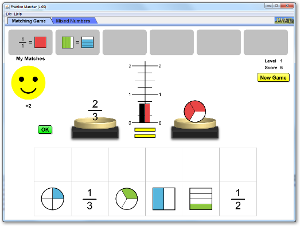# 分数匹配器下载嵌入代码 关闭 插入一张图片，当点击这个图片时启动仿真程序。回到HTML5 版本

• 分数
• 等值分数
• 带分数

### 示范 学习目标

• 查找匹配使用数字和图片找到匹配的分数。
• 使用不同的数字给出相同的分数。
• 在不同的图像模式中匹配不同的分数。
• 使用数字和模式比较分数。

### Standards Alignment

#### Common Core - Math

1.G.A.3
Partition circles and rectangles into two and four equal shares, describe the shares using the words halves, fourths, and quarters, and use the phrases half of, fourth of, and quarter of. Describe the whole as two of, or four of the shares. Understand for these examples that decomposing into more equal shares creates smaller shares.
2.G.A.2
Partition a rectangle into rows and columns of same-size squares and count to find the total number of them.
2.G.A.3
Partition circles and rectangles into two, three, or four equal shares, describe the shares using the words halves, thirds, half of, a third of, etc., and describe the whole as two halves, three thirds, four fourths. Recognize that equal shares of identical wholes need not have the same shape.
3.NF.A.1
Understand a fraction 1/b as the quantity formed by 1 part when a whole is partitioned into b equal parts; understand a fraction a/b as the quantity formed by a parts of size 1/b.
3.NF.A.2
Understand a fraction as a number on the number line; represent fractions on a number line diagram.
3.NF.A.2a
Represent a fraction 1/b on a number line diagram by defining the interval from 0 to 1 as the whole and partitioning it into b equal parts. Recognize that each part has size 1/b and that the endpoint of the part based at 0 locates the number 1/b on the number line.
3.NF.A.2b
Represent a fraction a/b on a number line diagram by marking off a lengths 1/b from 0. Recognize that the resulting interval has size a/b and that its endpoint locates the number a/b on the number line.
3.NF.A.3
Explain equivalence of fractions in special cases, and compare fractions by reasoning about their size.
3.NF.A.3a
Understand two fractions as equivalent (equal) if they are the same size, or the same point on a number line.
3.NF.A.3b
Recognize and generate simple equivalent fractions, e.g., 1/2 = 2/4, 4/6 = 2/3. Explain why the fractions are equivalent, e.g., by using a visual fraction model.

### 教师指南仿真程序控制概述,简化模型,学生视角的思考 ( PDF ).

### 教师提交的活动

Fraction Matcher GameTrish Loeblein 高中
K-5

Exploring Equivalence with Rational Numbers: Part 2 Extending to Mixed NumbersMichael Matassa 初中
K-5

Exploring Equivalence with Rational Numbers: Part 1 Fractions less than or equal to 1Michael Matassa 初中
K-5

Alignment of PhET sims with NGSSTrish Loeblein 高中 其它 生物学

How do PhET simulations fit in my middle school program?Sarah Borenstein 初中 其它 生物学

CCSS Math Alignment with SimsAmanda McGarry 高中

K-5

Rasyonel Sayılarda Denklik: Bölüm 2 Bileşik Kesrin Tam Sayılı Kesir Olarak Yazılması Sebnem Atabas (Translated from Michael Matassa's Exploring Equivalence with Rational Numbers: Part 2 Lesson Plan) 初中 指导

MS and HS TEK to Sim Alignment Elyse Zimmer 初中

Matching Fractions Bethany Konz K-5

Fraction Matcher Grades 3-5 Molly Martin K-5 指导 数学
Kinematics Wang Yunhe 高中 实验室 物理学
Fraction Matcher Lesson Plan Mariel Crystall K-5 实验室

분수-그림 연결 SIM 사용설명서 이화국(Wha Kuk Lee) K-5

Windows Macintosh Linux
Microsoft Windows
XP/Vista/7/8.1/10